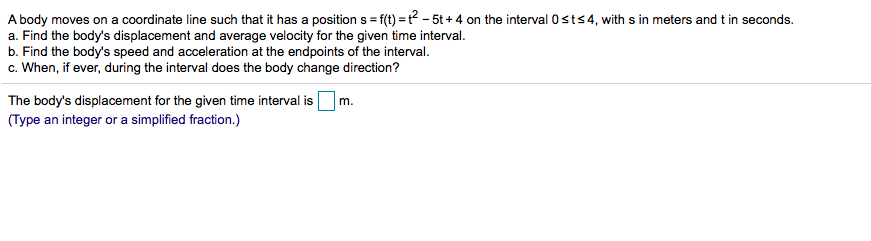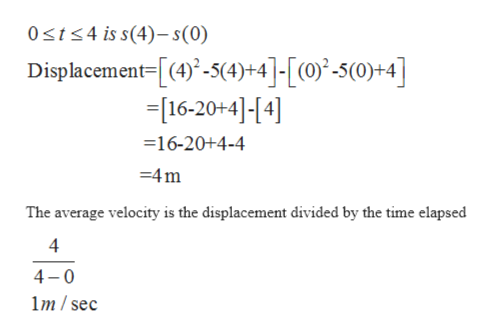A body moves on a coordinate line such that it has a position s f(t)t2-5t+4 on the interval 0sts4, with s in meters and t in seconds.a. Find the body's displacement and average velocity for the given time intervalb. Find the body's speed and acceleration at the endpoints of the intervalc. When, if ever, during the interval does the body change direction?The body's displacement for the given time interval ism.(Type an integer or a simplified fraction.)

Question

Can you help me step by step with this question pleasehelp_outlineImage TranscriptioncloseA body moves on a coordinate line such that it has a position s f(t)t2-5t+4 on the interval 0sts4, with s in meters and t in seconds. a. Find the body's displacement and average velocity for the given time interval b. Find the body's speed and acceleration at the endpoints of the interval c. When, if ever, during the interval does the body change direction? The body's displacement for the given time interval ism. (Type an integer or a simplified fraction.) fullscreen
Step 1

A body moves  on a coordinate  line such that  it has a  position  on the interval , with in  meters and t in seconds.

1. Find the body’s displacement and average velocity for the given time interval
2. Find the body’s speed and acceleration at the end point of the interval
3. When, if ever, during the interval does the body change direction?
Step 2

To find the displacement we need to find the s(b)- s(a) in the given interval

Step 3

The displacement for th...help_outlineImage Transcriptionclose0st4 is s(4)-s(0) Displacement=[(4)-5(4)+4]-[(0)2-5(0)+4] -[16-20+4]- 16-20+4-4 4m The average velocity is the displacement divided by the time elapsed 4 4 0 m/sec fullscreen

Want to see the full answer?

See Solution

Want to see this answer and more?

Our solutions are written by experts, many with advanced degrees, and available 24/7

See Solution
Tagged in

Derivative